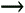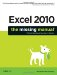Section 8.5. Advanced Statistics

Excel offers a smorgasbord of number- crunching tools; in fact, it has more than 50 advanced statistical functions that this chapter hasn't even mentioned. Many of these functions will appeal only to those who already understand concepts like standard deviation, theoretical distributions, and dimensionless Pearson product moment correlation coefficients (a subject of keen interest to all six people in the world who currently use such numbers ).

But even if you don't understand any of those concepts (and if you're still wondering whether your inner mathematician may yet emerge), Excel offers two advanced statistical functionsFORECAST( ) and TREND( )that give you a taste of all that awaits the advanced statistician. While both FORECAST( ) and TREND( ) rely on some complex, behind-the-scenes computations , using them is quite easy, even for non-specialists.

The FORECAST( ) and TREND( ) functions predict missing values based on existing data. These "missing" values may represent experimental data you didn't collect, or future sales numbers for dates that haven't occurred yet. Excel examines the data you have so far (the more the better), and uses a mathematical technique known as the method of least squares to generate a trend line. Excel then uses this trend line to generate the other values you've requested .

Both FORECAST( ) and TREND( ) work similarly and produce the same answers. The difference is that FORECAST( ) is designed to calculate a single value. The TREND( ) function, on the other hand, is an array function that gives you multiple values. It works a little like the FREQUENCY( ) function described on Section 8.2.8.

The FORECAST( ) and TREND( ) analyses work by trying to spot a relationship between two sets of numbers, designated as the x-axis and y-axis. To predict a new value in the FORECAST( ) function, for instance, you supply the function with a new x value. It then uses the known relationship between the x and y values to guess the corresponding y value. The syntax is as follows :

FORECAST(x, known_x_values, known_y_values)

For example, imagine you're trying to study the relationship between the number of product inquiries received during one month and the actual sales recorded in the next month. You have plenty of historical data, and you'd like to use that to predict how many sales you should expect next month (based on the number of inquiries you've received during the current month). The existing inquiry numbers are the first set of known values, so they make up the x-axis values (the cells A2:A9, as shown in Figure 8-9). The second set of known values is the actual recorded sales in the following month (the y-axis values, shown in cells B2:B9). You want to predict a new y-axis value for expected sales based on the current inquiries ( cell A12). Here's the final formula:

=FORECAST(A12,B2:B9,A2:A9)

Note: The FORECAST( ) and TREND( ) functions can always find some sort of relationship using your data. However, if your data doesn't have a real correlation, the values they predict will be meaningless. For example, the number of product inquiries might not be an effective predictor of future sales, especially when you consider other factors. Determining where correlations exist and what relationships are statistically significant is a much more subtle and complex issue than just using the FORECAST( ) and TREND( ) functions. An understanding of basic statistics will set you down the right path .Figure 8-9. This worksheet shows how you can forecast a future value based on a historical relationship by using the FORECAST( ) function. The example here predicts sales based on an analysis of the previous relationship between inquiries and gross sales.

You can also use the TREND( ) function to generate expected sales based on multiple inquiry numbers (as opposed to the single sales value that FORECAST( ) generates). Using the previous example, you could theoretically try to predict sales for the next six months. In truth, that approach doesn't really make sense because, while you have previous sales data to draw from, you don't know how many inquiries you'll receive in future months.

A better idea is to use TREND( ) to project future sales based on a slightly different set of data. You still use previous sales, but rather than using inquiries as your second known factor, you use the progression of time . In effect, you're asking TREND( ) if your sales over periods 1, 2, and 3 were 100, 150, and 200, what would sales look like over periods 4, 5, 6? Without further ado, here's what you need to generate new, predicted sales figures using the TREND( ) function:

1. Make sure your worksheet has all the data your TREND( ) formula needs.

As shown in Figure 8-10, you need three groups of numbers: known y-values (historical sales figures), known x-values (previous time periods), and the new x-values (known, new time periods). What you want the formula to figure out are the new y-values (that is, the sales figures).Figure 8-10. All signs point to a strong year. Based on the previous growth of sales, Excel cheerily predicts four more quarters of escalating growth. (The italicized cells are the ones that Excel has predicted.) Keep in mind, of course, that Excel generated these numbers using the pattern of the last two years . Various other factors could emerge that might make this trend line completely irrelevant.

2. Select the range of cells where you want to display the predicted values.

In this example, four new sales figures appear in cells C11:C14.

3. Enter the array formula using the TREND( ) function.

Here's the formula used in this example:

=TREND(C2:C9, A2:A9, A11:A14)

4. When you've finished typing in the formula, hit Ctrl+Shift+Enter.

You can't simply press Enter, as that will only enter the formula into the first selected cell. Instead, you need to press Ctrl+Shift+Enter to copy the array formula into all the result cells. You can tell that an array formula is at work by the curly braces {} in the formula bar, which Excel adds automatically.

Excel provides many more advanced statistical functions. For the full list, refer to the Statistical group in the Insert Function dialog box (select InsertFunction). Table 8-3 outlines some of the more commonly used functions in this group.

 Power User's Clinic An Advanced Engineering Toolkit When you turn on the Analysis ToolPak add-in (as explained on Section 7.2.4), you'll find that Excel creates a new category for some very specialized mathematical functions named Engineering. These functions tackle two primary problem areas: imaginary, or complex numbers (numbers that incorporate i , the square root -1); and number conversions between binary, decimal, and octal representations. If you need to use these functions for advanced mathematical spreadsheets, you'll find them quite understandable. On the other hand, if you don't know the difference between a complex conjugate and a vector, they probably won't be of much use. Excel doesn't corner the market on advanced mathematical functions. Many more specialized functions are available if you install add-ins from other vendors . And in Chapter 26, you'll learn how you can write the custom logic for your own functions using Visual Basic for Applications (VBA).

Table 8-3. Advanced Statistical Functions

Function

Syntax

Description

AVEDEV( )

AVEDEV(range)

Calculates the average deviation, which is the average of how much a series of data points deviate from the mean.

CORREL( )

CORREL(range1, range2)

Calculates the correlation coefficient between two sets of data, which is a measure of how the sets are related . The correlation coefficient is always between -1 and 1 (the closer to 1 the stronger the correlation, while 0 means no correlation at all).

COVAR( )

COVAR(range1, range2)

Calculates the covariance, which is the average of the products of deviation for each data point pair. Covariance is used to determine if a relationship exists between data sets.

LINEST( )

LINEST(known_y, known_x)

Calculates the best-fit line using the least squares method. This is an array function that returns both the slope and the y-intercept.

NORMDIST( )

NORMDIST(x, mean, standard_dev)

Calculates the normal cumulative distribution for a specified mean and standard deviation.

NORMINV( )

NORMINV(probability, mean, standard_deviation)

Calculates the inverse of the normal cumulative distribution for a specified mean and standard deviation.

SLOPE( )

SLOPE(known_y, known_x)

Calculates a regression line through the supplied data points, and returns its slope.

STDEV( )

STDEV(range)

Calculates the standard deviation of a data set. Blank and text cells are ignored.

STDEVA( )

STDEVA(range)

Calculates the standard deviation of a data set. Text cells are used as 0 values but blank cells are ignored.

VAR( )

VAR(range)

Estimates the variance of a sample population. Text cells are used as values but blank cells are ignored.

VARA( )

VARA(range)

Estimates the variance of a sample population. Text cells are used as values but blank cells are ignored.Excel 2010: The Missing Manual
ISBN: 1449382355
EAN: 2147483647
Year: 2003
Pages: 185

Similar book on Amazon